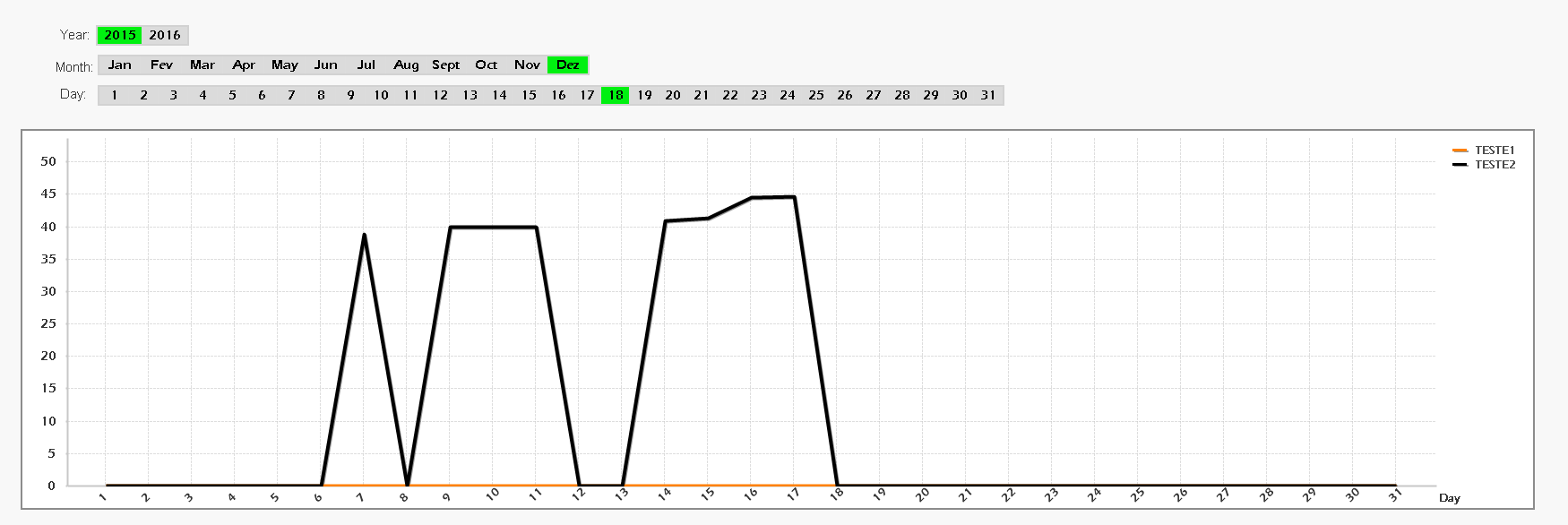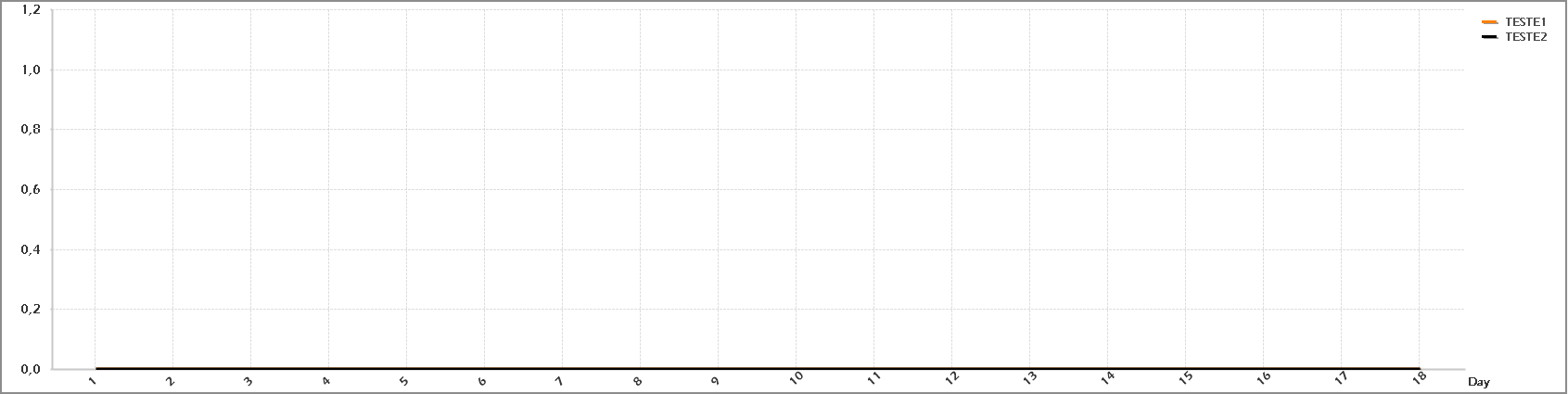QlikView App Development

Discussion Board for collaboration related to QlikView App Development.Not applicable

Qlikview - Get Max Selected Day of Month

Hi ExpertsI’ve the following situation:- I’ve the Year, Month and Day selected because I only show the evolution until the day selected (in this case is 18 of Decemeber)

- If you repair the Chart don’t do any calculation after the selected day (only returns 0s), and it’s fine, but it still showing the days after 18. But I can Hide Zeros because I still want to show the days provided before the day selected.

What I want is the chart Like that (this in a example from Excel without values only to show the goal). Basically I want to get only the days before the day selected even If it contains 0s:I'm using some conditional expressions to play with Month, Year and Day but I can't get the chart I wantHere are my expressions for Teste1 (I take from another post where Sunny T post a similar thing):

• Teste1
• Conditional 1:
• =GETSELECTEDCOUNT(YEAR) >= 1 and GETSELECTEDCOUNT(DAY) < 1
• Expression:
• =COUNT({<DATE = {"\$(='>=' & Date(YearStart((DATE))) & '<=' & Date(Max(DATE)))"},YEAR,MONTH, DAY>} TESTE1)

• Conditional 2:
• =GetSelectedCount(DAY) >= 1
• Expression:
• =COUNT({<DATE = {"\$(='>=' & Date(MonthStart((DATE))) & '<=' & Date(Max(DATE)))"},YEAR,MONTH, DAY>} TESTE1)

Thanks!!!!8 RepliesMVP

Re: Qlikview - Get Max Selected Day of Month

Maybe just add a field modifier for DAY:

=COUNT({<DATE = {"\$(='>=' & Date(MonthStart((DATE))) & '<=' & Date(Max(DATE)))"},YEAR,MONTH, DAY = {"<=\$(=Max(DAY))"} >} TESTE1)Not applicable

Re: Qlikview - Get Max Selected Day of Month

Swuehi, so many thanks!!!!!Not applicable

Re: Qlikview - Get Max Selected Day of Month

One more thing swuehl,

When I insert some more set analysis in the code I loose some days of my chart, imagine that:

=SUM({<DATE = {"\$(='>=' & Date(MonthStart((DATE))) & '<=' & Date(Max(DATE)))"},YEAR,MONTH,DAY = {"<=\$(=Max(DAY))"},FIELDX = {'X'}, DAY>} TESTE1)

I'm loosing some days of my month... Do you know why?MVP

Re: Qlikview - Get Max Selected Day of Month

Not sure, hard to answer without knowing your data model and your data values a bit more detailed.

Probably the FIELDX values have not relations to all possible DAY values.

Try maybe something like

=SUM({<DATE = {"\$(='>=' & Date(MonthStart((DATE))) & '<=' & Date(Max(DATE)))"},YEAR,MONTH,DAY = {"<=\$(=Max(DAY))"},FIELDX = {'X'}, DAY>} TESTE1)

+

SUM({<DAY = {"<=\$(=Max(DAY))"}>} 0)MVP

Re: Qlikview - Get Max Selected Day of Month

Why are there two set modifiers for field DAY in your expression? See the end of your set specification.Not applicable

Re: Qlikview - Get Max Selected Day of Month

I put the Day in the end to try to get the values that are losted... but probably is a problem related with my data modelNot applicable

Re: Qlikview - Get Max Selected Day of Month

Swuehl, one more time thank you very much!You answer was perfect!!! Do you know why it happens without the second SUM()?MVP

Re: Qlikview - Get Max Selected Day of Month

You should only use a single modifier per field in a single set modifier <....>, so as a general rule, only use a FIELD Name once between a set of <....>. So please remove the last DAY in your expression, this may also resolve your issue (even without the second sum).

Good catch by Peter.